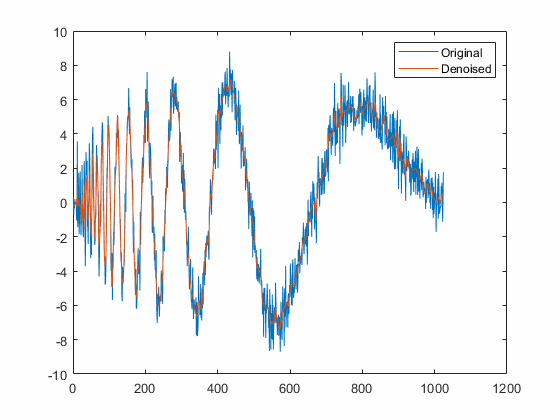Main Content

## Generate Code to Denoise a Signal

This example shows how to use MATLAB® Coder™ to generate executable code. The Wavelet Toolbox™ supports code generation for functions that support discrete wavelet transform (DWT), maximal overlap discrete wavelet transform (MODWT), wavelet packet transform (WPT), maximal overlap wavelet packet transform (MODWPT), and denoising workflows. This example requires a MATLAB Coder license.

Define a function that uses `wdenoise` to denoise a signal. You also specify the level to which to denoise the signal when you run the generated code.

1. From the MATLAB command prompt, create the file, `sigdenoise.m`.

`edit sigdenoise`
If you do not have permission to write to the current working folder, change the current folder to one that is writable.

2. Copy this `sigdenoise` function code into the `sigdenoise.m` file. Your file must include `%#codegen` to indicate that this function will generate code.

```function xdenoise = sigdenoise(x,level) %#codegen wname = 'sym4'; xdenoise = wdenoise(x,level,'Wavelet',wname,... 'DenoisingMethod','SURE',... 'ThresholdRule','soft',... 'NoiseEstimate','LevelIndependent'); end ```

3. Save the file.

4. At the MATLAB command line, use the `codegen` function to compile the `sigdenoise` function into a MEX file. You can use the `-o` option to specify the name of the executable. If you do not use the `-o` option, the generated MEX file has the same name as the original MATLAB file with `_mex` appended. You can include the `-report` option to generate a compilation report. This report shows the original MATLAB code and the associated files created during code generation. The `-args` option specifies the data types of the inputs required to run the generated code. In this case, a variable-size row vector and a scalar input are required.

`codegen sigdenoise.m -config:mex -args {coder.typeof(1,[1 inf]),0}`

5. At the MATLAB command line, run the generated code on noisy Doppler data and denoise it to level three. Compare the original and denoised signals.

```load noisdopp xden = sigdenoise_mex(noisdopp,3); plot([noisdopp',xden']) legend('Original','Denoised')```For a list of Wavelet Toolbox functions supported for code generation and associated limitations, see Code Generation Support, Usage Notes, and Limitations. For more information on code generation, see Get Started with MATLAB Coder (MATLAB Coder).

## SupportGet trial now